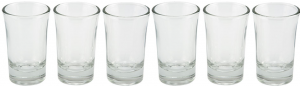Related Articles
Puzzle | Upside-Down Glasses
• Difficulty Level : Medium
• Last Updated : 22 Jan, 2018

There are n glasses on the table, all standing upside down. In one move, you are allowed to turn over exactly n – 1 of them. Determine all values of n for which all the glasses can be turned up in the minimum number of moves.There exists 2 cases, i.e, when the value of n is odd and when the value of n is even. If the value of n is odd, then there exists no solution.If the value of n is even, the problem can be solved in n moves, which is the minimum number of moves required.

If n is odd : As in one move,we are allowed to turn over exactly n – 1 of them.If n is odd then n – 1 i.e, the number of glasses turned over in one move, is even. Therefore, the parity of the number of glasses that are upside down will always remain odd, as it was in the initial position. Hence, the final position in which the number of upside down glasses is 0, which is even, cannot be reached.

If n is even : Let us assume that the glasses are numbered from 1 to n.If n is even, the problem can be solved by making the following move n times: turn over all the glasses except the ith glass, where i = 1, 2, . . . , n.
Here is an example of the algorithm’s application for n = 6. Let us denote the upside down glasses by 1’s and the others as 0’s. A glass that is not turned over on the next move is shown in bold.

If the value of i is 1, then turn over all the glasses except the 1st glass.
111111 –> 100000
Similarly, If the value of i is 2, then turn over all the glasses except the 2nd glass.
100000 –> 001111
Similarly, If the value of i is 3, then turn over all the glasses except the 3rd glass.
001111 –> 111000 and so on.
So, for n = 6, this will look like :

111111 –> 100000 –> 001111 –> 111000 –> 000011 –> 111110 –> 000000

Since any two consecutive moves will either have no impact on the state of the glasses or change the state of exactly two of them, no algorithm can solve the problem in fewer than n moves.

My Personal Notes arrow_drop_up
Recommended Articles
Page :